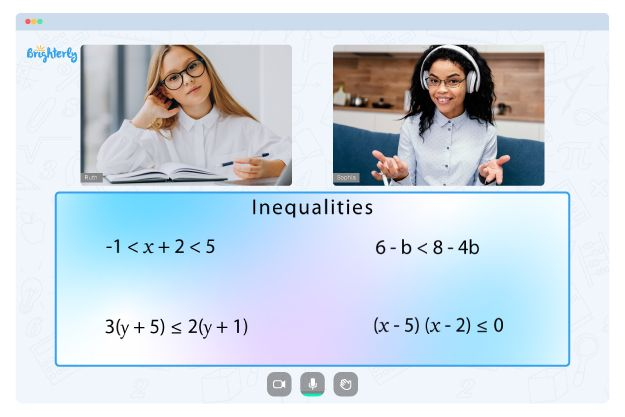Inequalities refer to balances such as greater than (>), less than (<), equal to (=), greater than or equal to (≥), and less than or equal to (≤). Mathematically, the inequalities represent the relationship between two expressions. So, how do you introduce inequalities to 7th graders? Keep reading to learn everything you should know about a 7th grade inequalities worksheet.

## Why Do Brighterly Tutors Use 7th Grade Inequalities Worksheets?

Brighterly is an online platform with educators who teach kids math using worksheets, games, and other exciting hands-on activities. While learning, tutors can recommend a 7th grade inequalities worksheet to help students realize the mathematical relation between two non-equal quantities.

Math for Kids

Is Your Child Struggling With Math?
1:1 Online Math TutoringWith an inequalities 7th grade worksheet, kids can conveniently learn the concept from the basics before moving on to the more complex topics. Also, a 7th grade inequalities worksheet with answers is available so that students can confirm the correctness of their solutions while working on the practice questions.

## How to Use 7th Grade Inequalities Worksheet in a Fun Way### 7th Grade Inequalities Worksheets PDF### 7th Grade Inequalities Worksheets PDF### 7th Grade Inequalities Worksheets PDF### 7th Grade Inequalities Worksheets PDF

Tutors use solving inequalities worksheet 7th grade to help students understand that math and inequality are everywhere. With the proper guide, it’s not hard to find examples of inequality if students keep their eyes open. So, instructors acknowledge that understanding the basics of inequalities is a necessary step before being able to use this knowledge in the real world.

Struggling with Inequalities?• Is your child having difficulties with understanding inequalities?
• An online tutor could provide the necessary help.

Does your child need additional support with mastering inequalities? Start lessons with an online tutor.